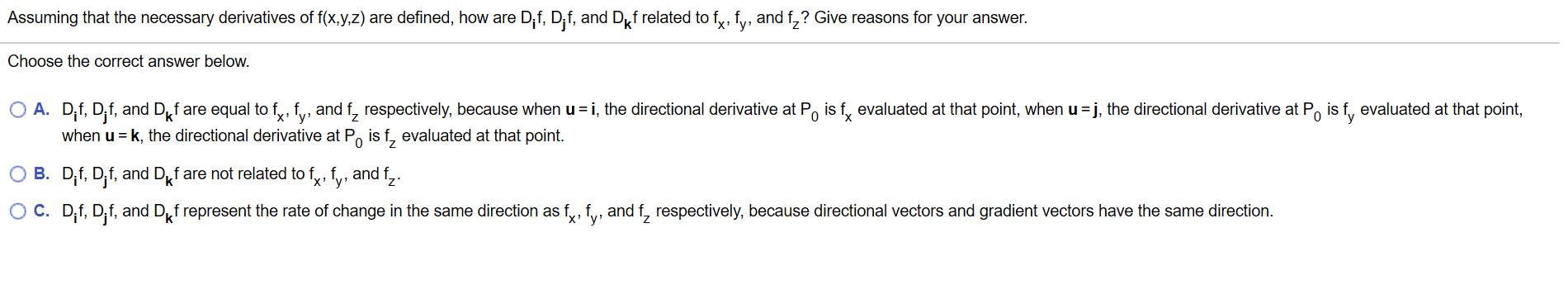# Assuming that the necessary derivatives of f(x,y,z) are defined, how are Dif, Dif, and Df related to f, fy, and f,? Give reasons for your answer.Choose the correct answer below.O A. D,f, D,f, and Df are equal to fy, fy, and f, respectively, because when u i, the directional derivative at Po is f, evaluated at that point, when u j, the directional derivative at Po is f, evaluated at that point,when u k, the directional derivative at Po is f, evaluated at that point0XO B. D,f, D,f, and Df are not related to fy, fv, and f,.O C. D,f, D,f, and Df represent the rate of change in the same direction as fy, f, and f, respectively, because directional vectors and gradient vectors have the same direction.

Question
67 views

How are the directional derivative and partial derivative related (more information in the image)help_outlineImage TranscriptioncloseAssuming that the necessary derivatives of f(x,y,z) are defined, how are Dif, Dif, and Df related to f, fy, and f,? Give reasons for your answer. Choose the correct answer below. O A. D,f, D,f, and Df are equal to fy, fy, and f, respectively, because when u i, the directional derivative at Po is f, evaluated at that point, when u j, the directional derivative at Po is f, evaluated at that point, when u k, the directional derivative at Po is f, evaluated at that point 0 X O B. D,f, D,f, and Df are not related to fy, fv, and f,. O C. D,f, D,f, and Df represent the rate of change in the same direction as fy, f, and f, respectively, because directional vectors and gradient vectors have the same direction. fullscreen
check_circle

Step 1

The correct answer is the first option i.e. option A.

Step 2

Each of the three co...

### Want to see the full answer?

See Solution

#### Want to see this answer and more?

Solutions are written by subject experts who are available 24/7. Questions are typically answered within 1 hour.*

See Solution
*Response times may vary by subject and question.
Tagged in

### Derivative